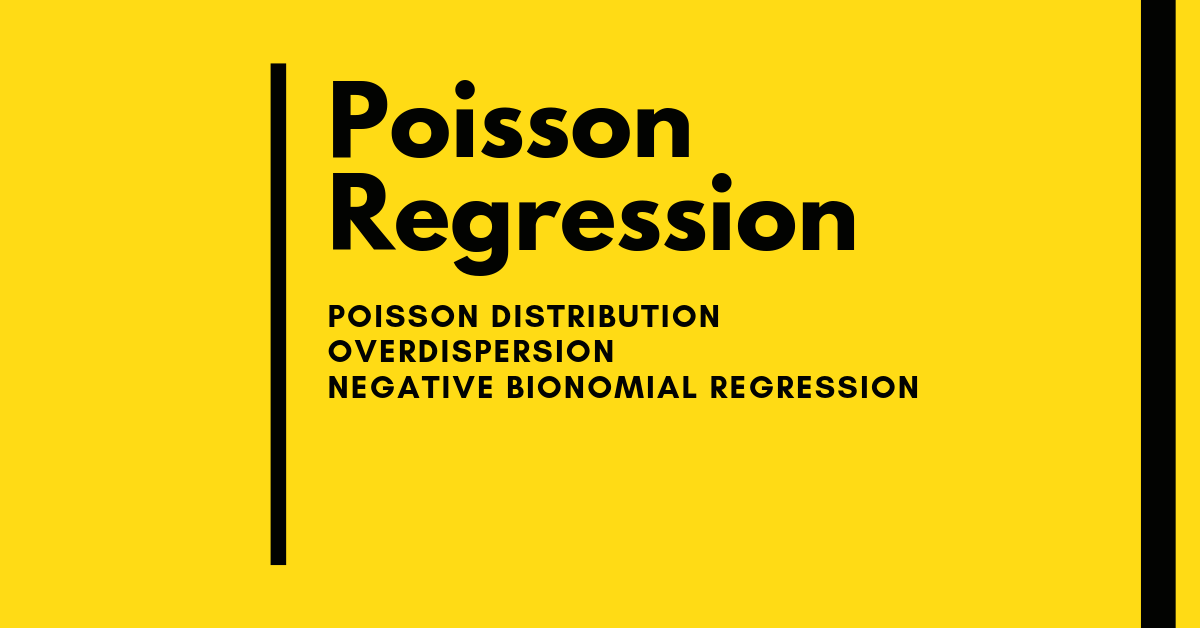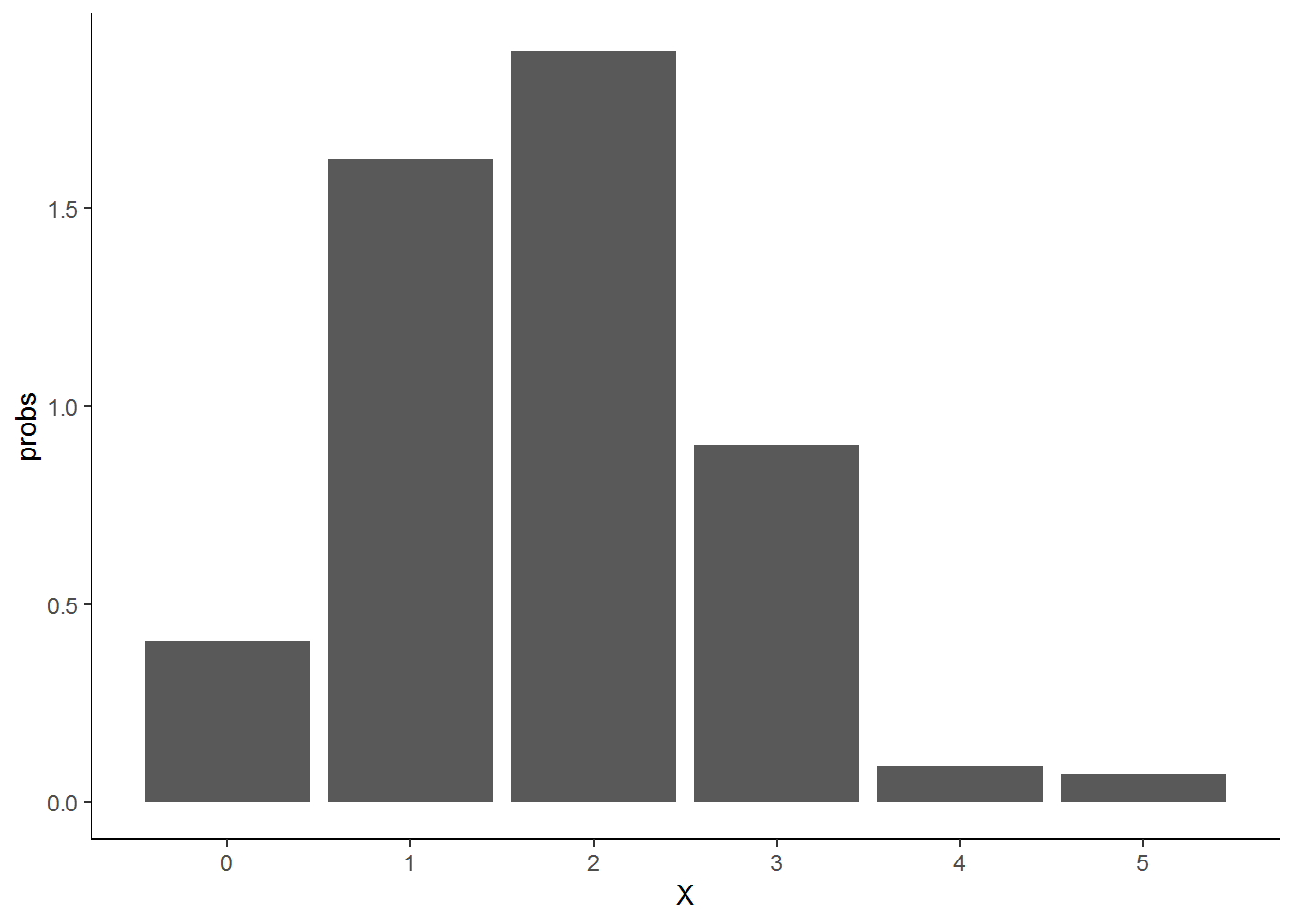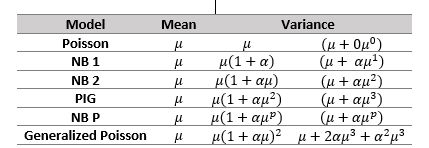# Poisson regression : Statistical models for counts and rates## What is a count ?

Count is a number which is discrete and non-negative. Discrete means a number which is a countable and distinct. For example, number of road accidents. Road accidents can be 2 or 852 but can not be 2.1 or 85.5. So its discrete i.e integer. This is different to numeric continuous variable (such as blood pressure 120.2,135.9) which modelled differently.

Count may also have contextual information i.e with time, area or length. Such as number of road accidents in given year, number of rain drops in square meter area. When the count is associated with any denominators, rate can be derived. For example, we observe 25 number of maternity claims from 850 women in year then the rate of claims will be 29 per 1000 women per year.

## Probability distribution(s) associated with the counts

First we need to clarify what is a distribution.The Distribution is the specification of probability associated with the value taken by random variable on random experiment. For example, in random experiment of counting number of rain drops in square meter area. The random variable is number of rain drops. Once the experiment has been performed, we count the drop which may be 10. The random variable X taken the value of 10.We do this experiment again and its 35. So random variable X has taken the value of 35.

Now if we know the average rate of some event $$[\lambda]$$ in which event happening indepdently in time. Then the number of event in any time period has poisson distribution.

For example, in neighborhood road, on avaerge 2 cars passing by every hour. We noted the number of cars passing by continuously for 24 hours. We may get following numbers

set.seed(125)
rpois(24,2)
##   3 0 1 1 5 5 2 1 2 2 0 3 1 0 2 2 2 4 2 3 1 3 3 1

We can derive the probability associated with each event and plot it with number of cars ( X axis ) and probability associated with it on Y axis

set.seed(125)
X <- rpois(24,2)
probs <- dpois(X,2)
require(ggplot2)

ggplot(data.frame(X,probs), aes(X,probs)) + geom_bar(stat = "identity") + scale_x_continuous(breaks=seq(0,5,by=1)) + theme_classic()require(dummies)
x <- sample(x=c("A","B"),size=100, replace=TRUE, prob=c(0.5,0.5))
linpred <- cbind(1, dummy(x)[, -1]) %*% c(0.2, 0.4)
y  <- exp(linpred)
df <- data.frame(x,y)
fit <- glm(y~x,family = poisson(), df)
summary(fit)
##
## Call:
## glm(formula = y ~ x, family = poisson(), data = df)
##
## Deviance Residuals:
##        Min          1Q      Median          3Q         Max
## -8.635e-09  -8.635e-09  -8.635e-09   0.000e+00   0.000e+00
##
## Coefficients:
##             Estimate Std. Error z value Pr(>|z|)
## (Intercept)   0.2000     0.1231   1.624   0.1043
## xB            0.4000     0.1646   2.430   0.0151 *
## ---
## Signif. codes:  0 '***' 0.001 '**' 0.01 '*' 0.05 '.' 0.1 ' ' 1
##
## (Dispersion parameter for poisson family taken to be 1)
##
##     Null deviance: 5.9609e+00  on 99  degrees of freedom
## Residual deviance: 4.0263e-15  on 98  degrees of freedom
## AIC: Inf
##
## Number of Fisher Scoring iterations: 4

The prime distribution of count is poisson. The other models are available to overcome two majors issues in counts : over-dispersion (or less often under-dispersion) and excess zeros. Or sometime zero is impossible value for the outcome ( such length of stay-no 0 length of stay), in this condition, zero truncated model is useful.

To overcome over or under dispersion:

• Negative Binomial distribution (NB)
• Poisson Inverse Gaussian (PIG)
• Generalized Negative Binomial Model
• Generalised Poisson
• Heterogeneous Negative Binomial model (NB-H)

To ovecome excess zero’s:

• zero-inflated Poisson (ZIP)
• zero-inflated negative binomial (ZINB)
• Hurdle model

To model outcome where 0 is impossible value

• zero truncated modelsFigure 1 Models for count data: mean-variance relationships and its parameterisation

### Poisson distribution

• single parameter
• mean $$[\mu]$$ is equal to variance $$[\mu]$$, also called dispersion
• in real life datasets, more often there will be higher variability or correlation than model allows (over-dispersion) which leads to biased standard errors ( so as misleading significance of covariate)

### Negative binomial distribution

• has additional dispersion parameter $$[\alpha]$$ to accommodate excess variability. when the dispersion parameter is zero, the model becomes Poisson
• can be traditional negative binomial (NB1) or quadratic negative binomial (NB2)
• Only correct over-dispersion ( not under-dispersion of poisson)
• form of Poisson-gamma mixture with dispersion parameter $$[\alpha]$$ has gamma distribution. Gamma distribution is very flexible in shape so most (not all) dispersed count data modelled well with NB
• It is possible that NB may not correct over-dispersion in a Poisson model

### Poisson Inverse Gaussian (PIG)

• Similler to negative binomial except the dispersion parameter $$[\alpha]$$ has inverse Gaussian distribution
• Available in R gamlass package

### Generalized Negative Binomial Model (GNB)

• The GNB parametrizes the exponent on the second term of the negative binomial variance.
• To get initial idea whether data is suited to NB1 or NB2 or NB-P

### Generalised Poissson Models

• Si miller to above models but dispersion parameter can take negative values which also correct under dispersion if present

### Heterogeneous Negative Binomail model (NB-H)

• Dispersion parameter can be associated with particular covariate which bring significant dispersion in the model.

### Exact poisson regression

for unbalanced and sparse count data

In count model log of count are modelled with respect to linear predictors. This is will make sure that predicted count will always be positive.

The expected percentage of zero counts on the basis of the Poisson model is under 1%. If mean of count response is 5 or below and some 30% of the count observations consist of zeros,ZIP or ZINB will be good choice.

Article is being updated …

Ref:

Hilbe, J. (2014). Modeling Count Data. Cambridge: Cambridge University Press.